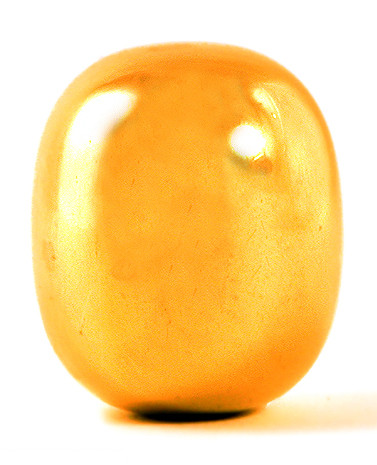# Supereggs, squigonometry, and squircles

The Depths of Wikipedia twitter account posted a screenshot about supereggs that’s popular at the moment. It says

there’s no way this is real. they must be making these words up

above a screenshot from the Wikipedia article on supereggs saying

The definition can be changed to have an equality rather than an inequality; this changes the superegg to being a surface of revolution rather than a solid.

I assume the Twitter account is having fun, not seriously suggesting that the terms are made up.

The terms “superegg” and “squircles” are whimsical but have been around for decades and have precise meanings. I hadn’t heard of “squigonometry,” but there are many variations on trigonometry that replace a circle with another curve, the best known example being hyperbolic trigonometry.

The equation for the volume of the superegg looked familiar but not quite right. It turns out the definition of superegg is not quite what I thought it was.Piet Hein coined the terms superellipse and superegg. The photo above is a brass superegg made by Piet Hein .

A superellipse is what mathematicians more commonly call a p-norm ball in two dimensions. I assumed that a superegg was a p-norm ball in three dimensions, but it’s not quite.

A unit p-norm ball in 3 dimensions has equationA superegg, however, has equationIf you slice a p-norm ball horizontally or vertically you get another p-norm ball. So in three dimensions, either a vertical or horizontal slice gives you a superellipse.

But a horizontal slice of a superegg is a circle while a vertical slice is a superellipse, which is not a circle unless p = 2. Said another way, supereggs are symmetric about the z-axis but p-norm balls are not.

I’ve left out one detail: superellipses and supereggs typically stretch one of the axes. So you’d replace x with x/k in the definition of a superellipse or replace z with z/k in the definition of a superegg. A squircle is a superellipse with the two axes equally, and typically p is set to 4 or a value near 4.